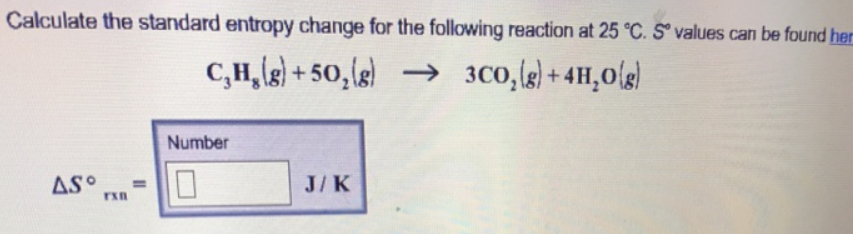# Problem: Calculate the standard entropy change for the following reaction at 25 °C. S° values can be found here.C3H8 (g) + 5O2 (g) → 3CO2 (g) + 4H2O (g)

###### FREE Expert Solution
89% (42 ratings)###### Problem Details

Calculate the standard entropy change for the following reaction at 25 °C. S° values can be found here.

C3H8 (g) + 5O2 (g) → 3CO2 (g) + 4H2O (g)Frequently Asked Questions

What scientific concept do you need to know in order to solve this problem?

Our tutors have indicated that to solve this problem you will need to apply the Entropy concept. You can view video lessons to learn Entropy. Or if you need more Entropy practice, you can also practice Entropy practice problems.

What professor is this problem relevant for?

Based on our data, we think this problem is relevant for Professor Ghosh's class at UCSD.